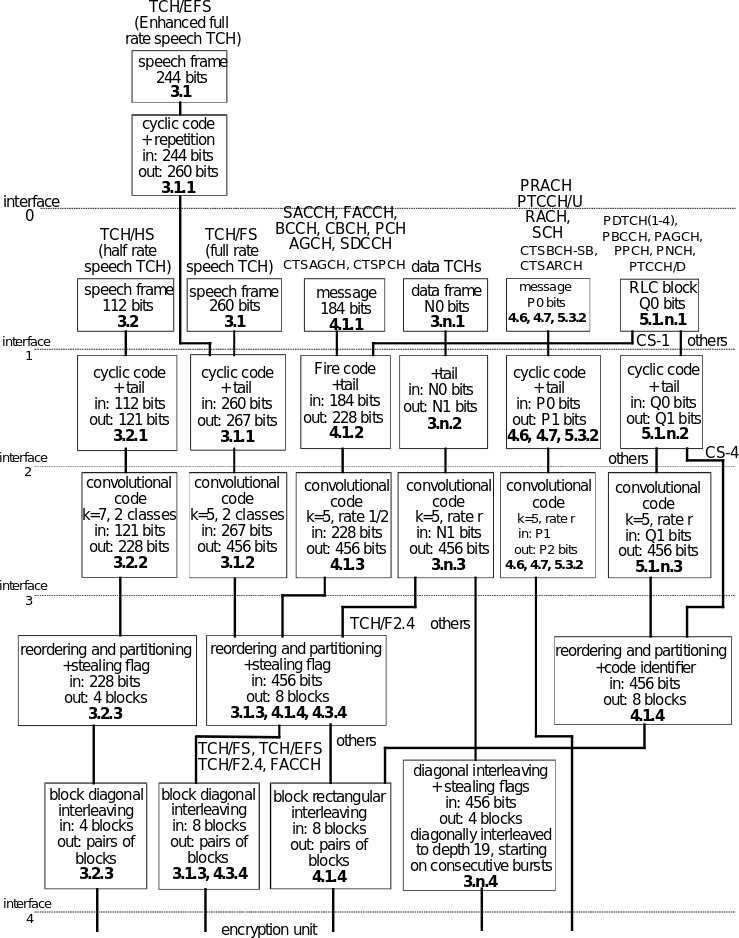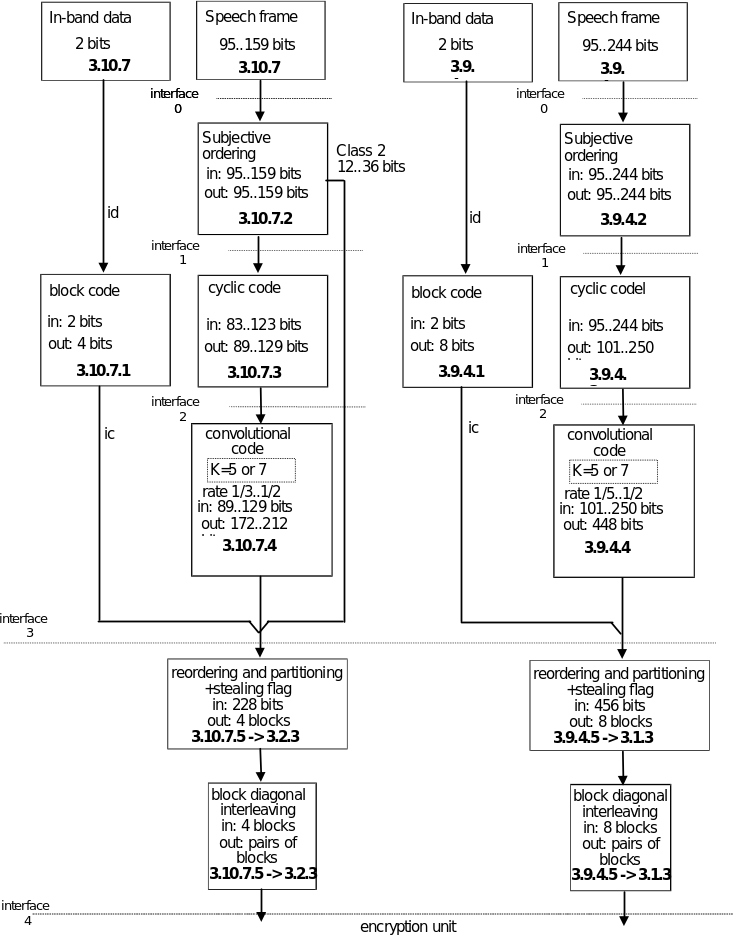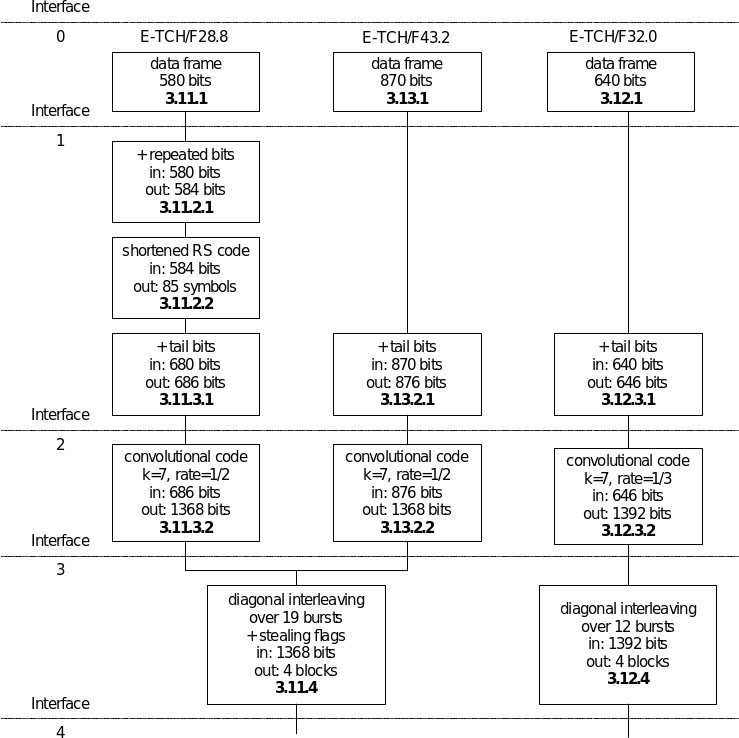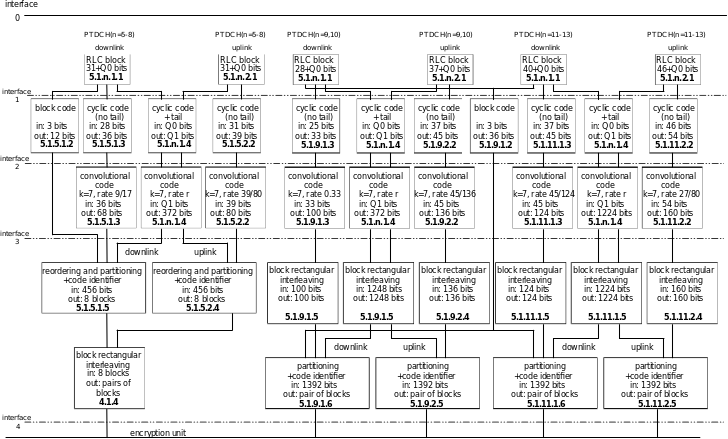## 2 General

05.033GPPChannel codingRelease 1999TS

## 2.1 General organization

Each channel has its own coding and interleaving scheme. However, the channel coding and interleaving is organized in such a way as to allow, as much as possible, a unified decoder structure.

Each channel uses the following sequence and order of operations:

‑ the information bits are coded with a systematic block code, building words of information + parity bits;

‑ these information + parity bits are encoded with a convolutional code, building the coded bits;

‑ reordering and interleaving the coded bits, and adding a stealing flag, gives the interleaved bits.

All these operations are made block by block, the size of which depends on the channel. However, most of the channels use a block of 456 coded bits which is interleaved and mapped onto bursts in a very similar way for all of them. Figures 1a and 1b give a diagram showing the general structure of the channel coding.

This block of 456 coded bits is the basic structure of the channel coding scheme. In the case of full rate speech TCH, this block carries the information of one speech frame. In case of control channels, it carries one message.

In the case of half rate speech TCH, the information of one speech frame is carried in a block of 228 coded bits.

In the case of the Enhanced full rate speech the information bits coming out of the source codec first go through a preliminary channel coding. then the channel coding as described above takes place.

In the case of a packet switched channel the block of 456 or 1384 coded bits carries one radio block.

In the case of E-TCH/F28.8 or E-TCH/F43.2, the block of 1368 coded bits (456 coded symbols) carries one radio block. In the case of E-TCH/F32.0, the block of 1392 coded bits (464 coded symbols) carries one radio block.

In the case of FACCH, a coded message block of 456 bits is divided into eight sub‑blocks. The first four sub‑blocks are sent by stealing the even numbered bits of four timeslots in consecutive frames used for the TCH. The other four sub‑blocks are sent by stealing the odd numbered bits of the relevant timeslot in four consecutive used frames delayed 2 or 4 frames relative to the first frame. Along with each block of 456 coded bits there is, in addition, a stealing flag (8 bits), indicating whether the block belongs to the TCH or to the FACCH. In the case of SACCH, BCCH, CCCH or CTSCCH, this stealing flag is dummy. In the case of a packet switched channel, these bits are used to indicate the coding scheme used.

In the case of E-FACCH/F, a coded message block of 456 bits is divided into four sub-blocks. The four sub-blocks are sent by stealing all symbols of four timeslots in consecutive frames used for the E-TCH and using GMSK modulation. The indication of the E-FACCH/F is based on the identification of the modulation. Along with each block of 456 coded bits there is, in addition, a stealing flag (8 bits), indicating whether the block belongs to the E-FACCH, FACCH or TCH.

Some cases do not fit in the general organization, and use short blocks of coded bits which are sent completely in one timeslot. They are the random access messages of:

– the RACH;

– or PRACH and CPRACH;

on uplink and the synchronization information broadcast on the SCH or CSCH on the downlink. In CTS, they are the access request message of the CTSARCH on uplink and the information broadcast on the CTSBCH-SB on downlink.Figure 1a: Channel Coding and Interleaving Organization

In each box, the last line indicates the chapter defining the function. In the case of RACH, P0 = 8 and P1 = 18; in the case of SCH, CSCH, CTSBCH-SB and CTSARCH, P0 = 25 and P1 = 39. In the case of data TCHs, N0, N1 and n depend on the type of data TCH.

Interfaces:

1) information bits (d);

2) information + parity + tail bits (u);

3) coded bits (c);

4) interleaved bits (e).

TCH/AHS TCH/AFSFigure 1b: Channel Coding and Interleaving Organization, adaptive multi-rate speech

In each box, the last line indicates the chapter defining the function.

Interfaces:

0) speech bits from the speech encoder (s);

1) reordered speech bits (d);

2) speech + parity + tail bits (u);

3) coded bits (c);

4) interleaved bits (e).Figure 2a: Channel Coding and Interleaving Organization for ECSD 8-PSK modulated signals

In each box, the last line indicates the chapter defining the function.Figure 2b: Channel Coding and Interleaving Organization for EGPRS Packet Data Channels

In each box, the last line indicates the chapter defining the function.

## 2.2 Naming Convention

For ease of understanding a naming convention for bits is given for use throughout the technical specification:

‑ General naming:

"k" and "j" for numbering of bits in data blocks and bursts;

"Kx" gives the amount of bits in one block, where "x" refers to the data type;

"n" is used for numbering of delivered data blocks where;

"N" marks a certain data block;

"B" is used for numbering of bursts or blocks where;

"B0" marks the first burst or block carrying bits from the data block with n = 0 (first data block in the transmission).

‑ Data delivered to the preliminary channel encoding unit (for EFR only):

s(k) for k = 1…, Ks

‑ Data delivered by the preliminary channel encoding unit (for EFR only) before bits rearrangement

w(k) for k = 1…, Kw

‑ Data bits delivered to the encoding unit (interface 1 in figure 1):

d(k) for k = 0,1,…,Kd‑1

‑ Data symbols delivered to the encoding unit:

D(k) for k = 0,1,…,KD‑1

– Input in-band data bits (for TCH/AMR only):

id(k) for k = 0, 1

– Encoded in-band data bits (for TCH/AMR only):

ic(k) for k = 0, 1,…,3 TCH/AHS speech frames or

k = 0, 1,…,7 TCH/AFS speech frames or

k = 0, 1,…,15 TCH/AMR, SID frames

‑ Code identifying the used coding scheme (for packet switched channels only):

q(k) for k = 0,1,…, 7

‑ Data bits after the first encoding step (block code, cyclic code; interface 2 in figure 1):

u(k) for k = 0,1,…,Ku‑1

– Data symbols after the first encoding step (block code):

U(k) for k = 0,1,…,KU‑1

– Data put into the shift register of the convolutional code and calculated from the data bits u(k) and the feedback bits in recursive systematic convolutional codes

r(k) for k= 0,1,…, Kr‑1

‑ Data after the second encoding step (convolutional code ; interface 3 in figure 1):

c(n,k) or c(k) for k = 0,1,…,Kc‑1

n = 0,1,…,N,N+1,…

‑ Interleaved data bits:

i(B,k) for k = 0,1,…,Ki‑1

B = B0, B0+1,….

‑ Interleaved data symbols:

I(B,k) for k = 0,1,…,KI‑1

B = B0, B0+1,….

‑ Bits in one burst (interface 4 in figure 1):

e(B,k) for k = 0,1,…,114,115

B = B0,B0+1,…

‑ Symbols in one burst (interface 4 in figure 2):

E(B,k) for k = 0,1,…,114,115

B = B0,B0+1,…

‑ E-IACCH messages delivered to the block coding of inband signalling (for ECSD only):

im(k) or im(n,k)

for k = 0,1,2

n = 0,1,…,N,N+1,…

‑ E-IACCH bits delivered to the mapping on one burst (for ECSD only):

ib(B,k) for k = 0,1,…,5

B = B0, B0+1,….

‑ E-IACCH symbols in one burst (for ECSD only):

HL(B) and HU(B)

for B = B0, B0+1,….